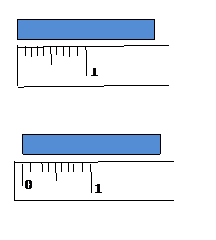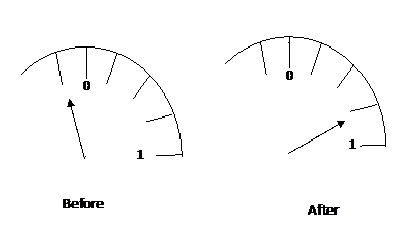Custom Search
If you want to discuss more on this or other issues related to Physics, feel free to leave a message on my Facebook page.

## Tuesday, December 22, 2009

### What is a zero error?

We have seen in a previous post what an error is. I am now going to talk about the zero error.

As the name suggest the error has a relation with the zero mark on a scale.

As you can see in fig 1 and fig 2 the scale on a measuring instrument can be either straight as on a meter rule or circular as on an ammeter.Fig 1Fig 2

Straight scale

Now when you measure using such instruments it is necessary for you to pay particular attention to the zero mark. If you are using a metre rule then one end of the object must be placed on the zero mark as shown in fig 3 below.Fig 3

However some measuring instruments have the the zero mark that start slightly inside like in the second diagram in fig 3 above. Be careful.

Now if the object is not place on the zero mark as shown in fig 4 below then a zero error is committed.Fig 4

Can you read the scale and tell me what is the length of the object?

Now as you can see the  object is not placed starting on the zero mark. It is place on the o.2 cm mark. This 0.2 cm mark is the magnitude of the error. As you can also see the object’s other end is on the 2 cm mark. Since the other end of the object is not place on the zero mark, the length obtained will be greater that the true value.

Hence the length of the ruler = 2.0 – 0.2 = 1.8 cm

It is important to identify the magnitude of the error and then to  remove it from the reading with the error to get the true reading.

Circular scale

When there is a circular scale, there is always a pointer like you can see on an ammeter or voltmeter. Now if you want to have the correct reading it is important for the pointer to be on the zero mark before it is used. If the pointer is not initially on the zero mark then a zero error would be committed.Fig 5                                                                      Fig 6

In fig 5 above you can clearly see that the pointer is on the zero mark before use, and as a result there is no zero error and the correct reading from fig 6 is 0.8.

Let us look at two examples where the pointer is not initially on the zero mark.Fig 7                                                                            Fig 8

In fig 7 the pointer is not on the zero mark before use. So in this case the solution is to adjust the pointer until the error is removed. If that is not possible then the magnitude of the error needs to be determined. In our example the the magnitude of the error is 0.3. Hence all measurement taken with this apparatus will be greater by 0.3. After the meter is used the reading is o.8. Then adjusted for the error

The true value = 0.8 – 0.3 =0.5Fig 9                                                         Fig 10

In fig 9 the pointer is on the left side of the zero mark. The magnitude of the error is 0.2. But as you can guess when the meter is in used the reading obtained will be less than the true value. Hence the reading from the meter in fig 10 is smaller than the true value and as a result the magnitude of the error must be added to the reading. Hence

The true value = 0.8 + 0.2  = 1.0

1.2.3.give a definition
for it please in one sentence

4.Zero error is the error a measuring instrument is reading when it should be on the Zero mark

5.A zero error arises when the measuring instrument does not start from exactly zero.

6.7.8.9.10.